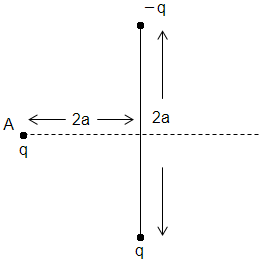Figure shows a ball having a charge q fixed at a point $\mathrm{A}$. Two identical balls having charges +q and –q and mass ‘m’ each are attached to the ends of a light rod of length $2\mathrm{a}$. The rod is free to rotate about a fixed axis perpendicular to the plane of the paper and passing through the mid-point of the rod. The system is released from the situation shown in figure. Find the angular velocity of the rod when the rod becomes horizontal.1.

2.

3.

4.